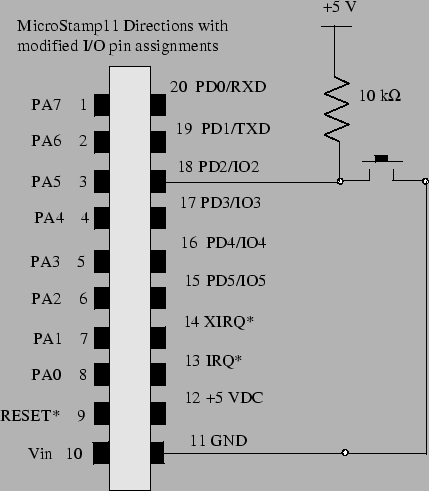Next: Resistive and Capacitive Loads Up: Analog and Digital Interfacing Previous: Input/Output Ports

## Mechanical Switches

This section shows how you interface the MicroStamp11 to a mechanical switch. To read the logical state of a pin, you must first make sure that the pin's direction state is set to "input". After that you must provide a valid TTL-voltage logic level of zero or 5 volts to the input pin. You can then look at the appropriate bit in the port variable (PORTA or PORTD) to hafve the program read the pin's logical state.

Remember that for PORTD you set the pin's direction state by setting the appropriate bit in the DDRDregister. Only two of the pins on PORTA are bidirectional and their direction states are set by the bits DDRA7 and DDRA3 in hardware register PACTL. So you can only use pins PA0-PA3 and PA7 as input pins.

So how does one supply a valid logical voltage level to the input pin? One might suppose that the circuit shown on the left hand side of figure 16 would work. But this circuit isn't a good design. The reason why this particular circuit won't work well can be explained as follows. First let's assume that the switch is closed. At this point the input pin will have a specified amount of charge sitting 5 volts away from ground. To keep the charge from draining away, the input pin, is essentially an open circuit. So if we open the switch, there is still all of this charge sitting on the input pin. We need to give that charge somewhere to go when we open the switch and this is done by connecting a resistor to either the +5 volt supply or to ground. This modified and much better circuit is shown in the right-hand drawing of figure 16.With the modified circuit shown in figure 16, we see that when the switch is closed, then we have a small current going through the 10 k-ohm resistor and the potential between the input pin and GND is 5 volts. When the switch is opened, all of the charge left on the input pin will drain way to ground through this resistor and the potential drop at the input pin is pulled-down to ground. So we refer to the resistor as a pull down resistor.

The preceding discussion addresses the electrical connection to the pin, but we still have a mechanical problem. When two contacts of the switch hit together, they tend to bounce off of each other a few times before settling down. Most mechanical switches have this bounce problem. You have probably never noticed this bouncing because it only lasts 1/100th of a second. The problem, however, is that the MicroStamp11 is fast enough to see each bounce as a separate hit.

The solution to this problem is called debouncing. Debouncing may be done in several ways. One may, for instance, use special input circuitry on the switch. It is, however, easier for us to do the debouncing in software. In particular, we simply need to deactivate the switch for a specified length of time after the first contact is made. This approach to software debouncing is what you should use.

In the following code segment we assume that PD3 is the logical name for bit 4 in PORTD. We assume that _Time is a global integer variable that is incremented every 1 millisecond by an appropriate timer interrupt handler. So the following code segment will debounce a button connected to PD3.

  DDRD &= ~PD3;
while((PORTD & PD3)==0);
_Time=0;
while( _Time < 10){};

The preceding code segment consists of four lines. The first line sets the input direction of bit PD3 on PORTD to input. The second line waits until bit PD3 goes high. Once this bit is high, we reset the global timer variable _Time to zero. Since we know that the kernel increments this variable every millisecond, we simply wait until this variable is greater than or equal to 10 (the last line of code). This code segment, therefore, forces us to wait 10 milliseconds after sensing the pin state going high. The idea is that any mechanical bouncing in the switch will be over after this 10 millisecond period.Next: Resistive and Capacitive Loads Up: Analog and Digital Interfacing Previous: Input/Output Ports
Bill Goodwine 2002-09-29